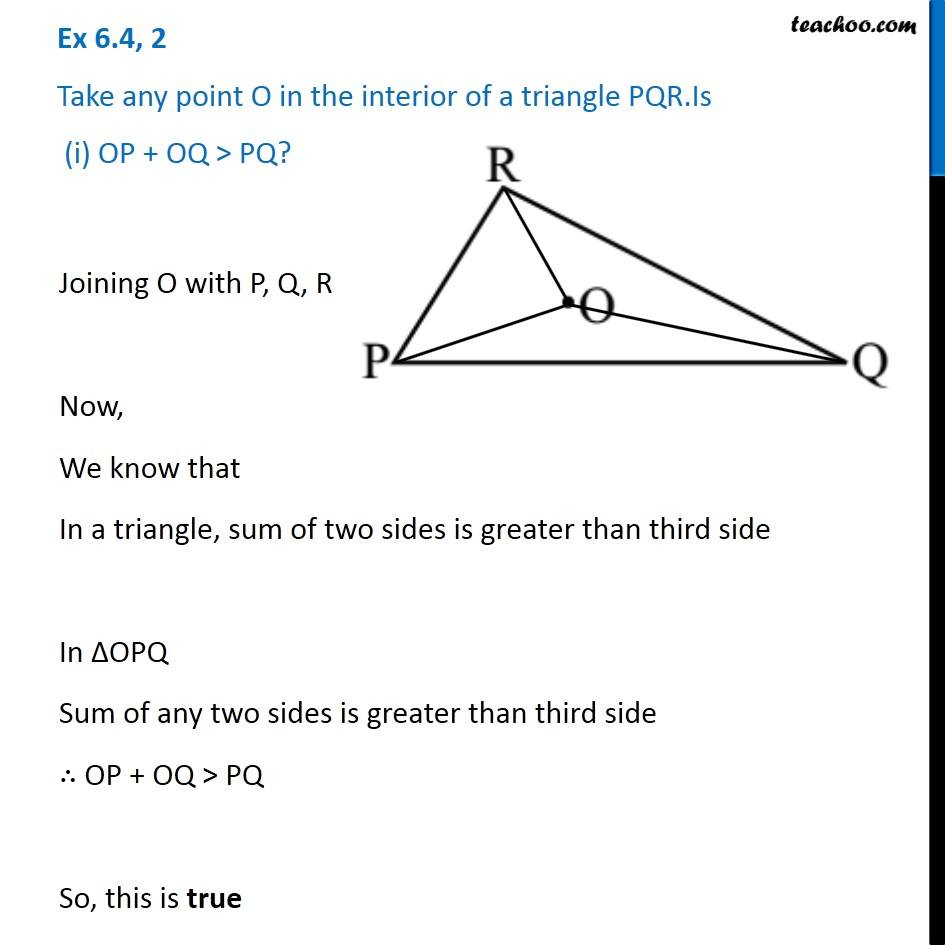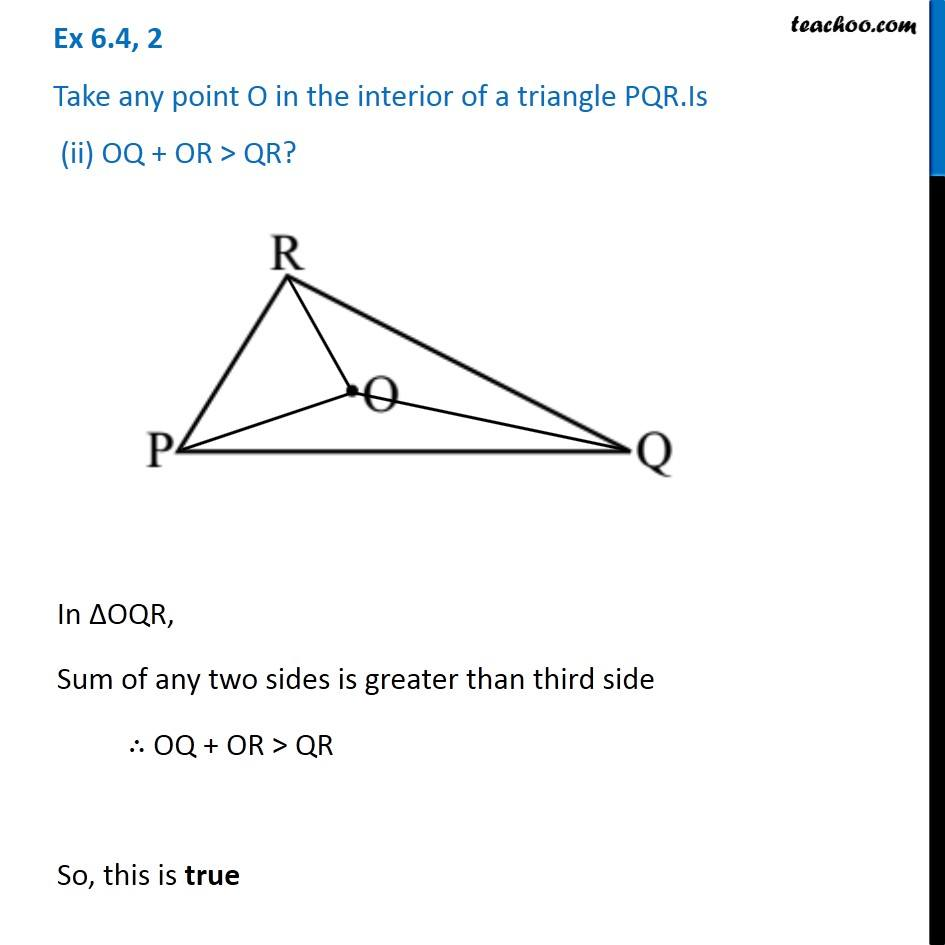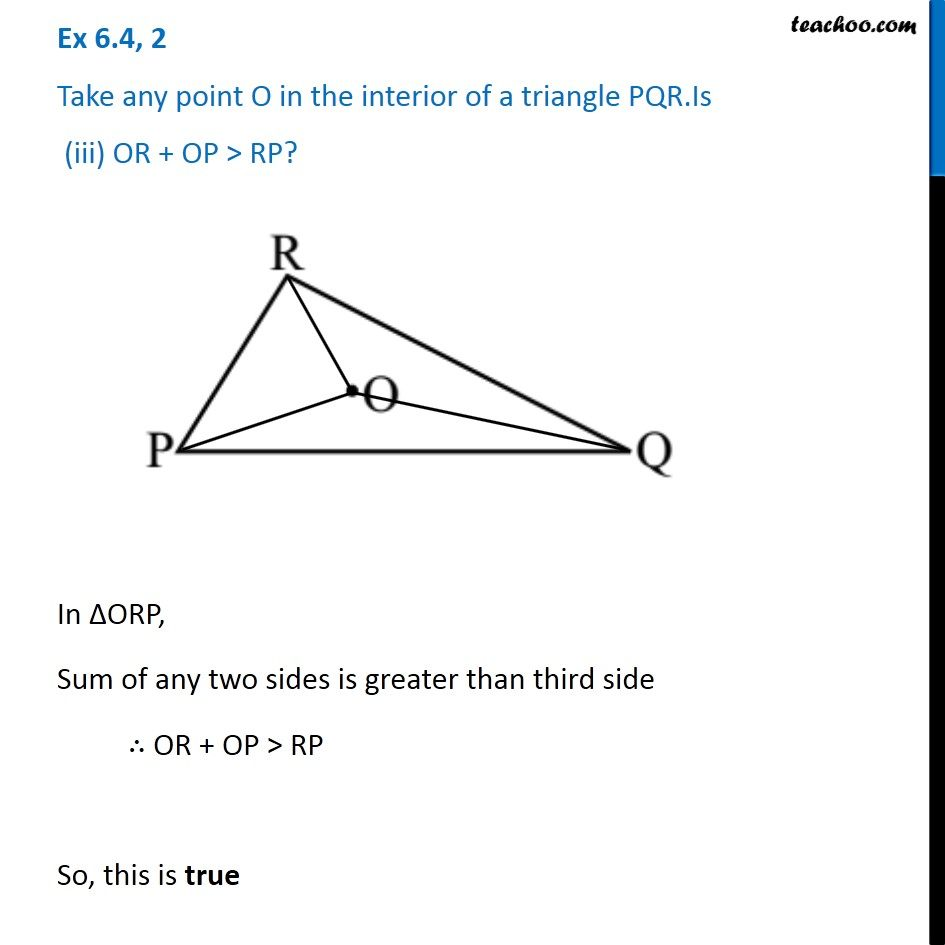1. Chapter 6 Class 7 Triangle and its Properties
2. Serial order wise
3. Ex 6.4

Transcript

Ex 6.4, 2 Take any point O in the interior of a triangle PQR.Is (i) OP + OQ > PQ?Joining O with P, Q, R Now, We know that In a triangle, sum of two sides is greater than third side In ∆OPQ Sum of any two sides is greater than third side ∴ OP + OQ > PQ So, this is true Ex 6.4, 2 Take any point O in the interior of a triangle PQR.Is (ii) OQ + OR > QR?In ∆OQR, Sum of any two sides is greater than third side ∴ OQ + OR > QR So, this is true Ex 6.4, 2 Take any point O in the interior of a triangle PQR.Is (iii) OR + OP > RP?In ∆ORP, Sum of any two sides is greater than third side ∴ OR + OP > RP So, this is true